Courses
Courses for Kids
Free study material
Offline Centres
MoreLast updated date: 29th Nov 2023
Total views: 380.4k
Views today: 9.80k

# Write number of edges, faces and vertices of cube, cuboid, cone, cylinder, sphere, triangular pyramid, rectangular and prism.Verified
380.4k+ views
Hint: In order to solve this problem make the diagram of each and every figure and recognize its number of edges, faces and vertices.

$\Rightarrow$Cube :-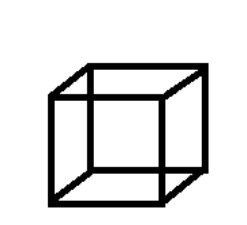In Maths or in Geometry, a Cube is a solid three-dimensional figure, which has 6 square faces, 8 vertices and 12 edges. Number of edges=12
Number of faces=6
Number of vertices=8

$\Rightarrow$Cuboid :-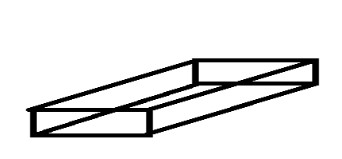The difference between cube and cuboid is, in a cube all the edges are of the same length and all the faces are square but in the cuboid all the faces are not square there may be rectangles as well.
Number of edges=12
Number of faces=6
Number of vertices=8
$\Rightarrow$Cone:-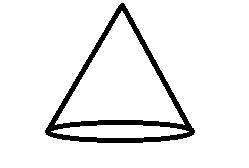The above diagram is of cones. A cone is a three-dimensional shape in geometry that narrows smoothly from a flat base (usually circular base) to a point(which forms an axis to the centre of base) called the apex or vertex.
Number of edges=0
Number of faces=1
Number of vertices=0
$\Rightarrow$Cylinder:-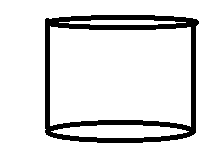The above shown diagram is of Cylinder. Cylinder is one of the basic shapes, in Mathematics, which has two parallel circular bases at a distance (called height of cylinder).
Number of edges=0
Number of faces=2
Number of vertices=0
$\Rightarrow$Sphere :-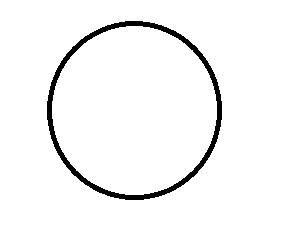The above shown diagram is of a sphere that is the same as the shape of a football. A sphere is an object that is an absolutely round geometrical shape in three-dimensional space.
Number of edges=0
Number of faces=0
Number of vertices=0
$\Rightarrow$Triangular Pyramid :-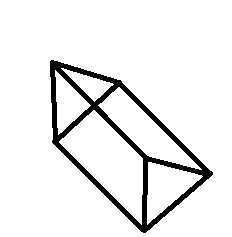The above shown diagram is of triangular prism. A triangular prism is a polyhedron, made up of two triangular bases and three rectangular sides. By the definition, the two triangular bases are parallel and congruent to each other.
Number of edges=9
Number of faces=5
Number of vertices=6
$\Rightarrow$Rectangular Prism:-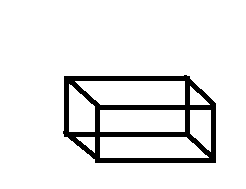A rectangular prism is a three dimensional shape. It has six faces, and all the faces of the prism are rectangles. Both the bases of a rectangular prism must be a rectangle. Also, the other lateral faces will be rectangles. It is also called a cuboid.
Number of edges=12
Number of faces=6
Number of vertices=8

Note: Whenever you face such type of problems draw the diagrams and count the number of edges, faces and vertices with the help of diagram. Proceeding like this will solve your problem and will give you the right answer.